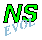## Bessel monopole encounters a north-south ridge f0=-3: southern hemisphere

The simulation discussed on the pabe Bessel monopole encounters a north-south ridge is with f0=0 in the Coriolis paramter. This means that the ridge is located at the equator. For non-zero f0-values, the monopole's evolution can be quite different, depending on the f0-value.

Consider the same initial situation as on Bessel monopole encounters a north-south ridge but now with f0=-3, which means that the ridge is on the southern hemisphere.

For all graphs: positive contours (0.1 to 2.0 at an interval of 0.1) are drawn solid, negative contours (-0.1 to -2.0 at an interval of -0.1) are drawn dashed, and the zero contour is dotted. Since the maximum of vorticity at the centre of the monopole is much larger than 2.0, the vortex looks like a "hole".
Note that the domain measures 20 by 20 length units -- the graphs show only the central part of this domain.

Turning on the time evolution means that the monopole moves to the north-west, due to the beta-effect, and it encounters the ridge. Due to the strong topography effect, there is now a creation of much stronger relative vorticity over the ridge and the monopole is repelled (rebounded) by the ridge:

The beta-effect makes that the monopole moves towards the ridge, but there is so much relative vorticity created at the ridge that the monopole cannot reach the foot of the ridge: together with the ridge-induced negative vorticity it forms an a-symmetric dipolar vortex which moves along a curved path away from the ridge.

The monopole manages to get rid of the extra vorticity and tries again to reach the ridge, but in vain: the vorticity induced by the ridge is too strong, as this graph shows:

Trajectory of the tracer (red) initially placed at the maximum of vorticity and of that maximum of vorticity (blue), both drawn until T=50.
The green line shows the monopole's trajectory in the shown part of the domain in the absence of the topography.

This MPEG movie (0.5 Mb; 101 frames) featuring the evolution from T=0 until T=50, shows far more clearly the effect of the ridge on the monopole

The background for this enormous difference in evolution between a run with f0=0 and the above one with f0=-3 is the much larger deformation of the contours of potential vorticity by the ridge for f0=-3:

For f0=-3 the monopole is completely surrounded by negative potential vorticity and the gradient of potential vorticity at the ridge is very strong, too strong for the monopole to even start climbing the ridge.

For f0=+3 the gradient of potential vorticity at the ridge is also very strong, but the effect is very different.

Note:
If the ridge is a trough, i.e. if h_max<0, then the effect shown above -- the destruction of the vortex by the topography -- occurs at negative f0.

```
```

For details on simulations with different f0-values and heights, see:
Van Geffen, J.H.G.M. and Davies P.A.: 1998,
"A monopolar vortex encounters a north-south ridge or trough,"
Fluid Dynamics Research, in press.

The evolution of the vorticity distribution is computed with a Finite Difference Method which solves the two-dimensional vorticity (Navier-Stokes) equation. Time and distances are given in dimensionless units.

===> Some details on the computation presented on this page for those who are interested.